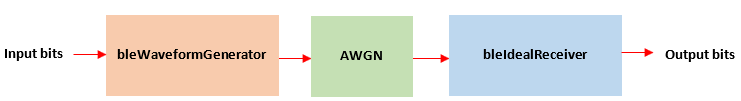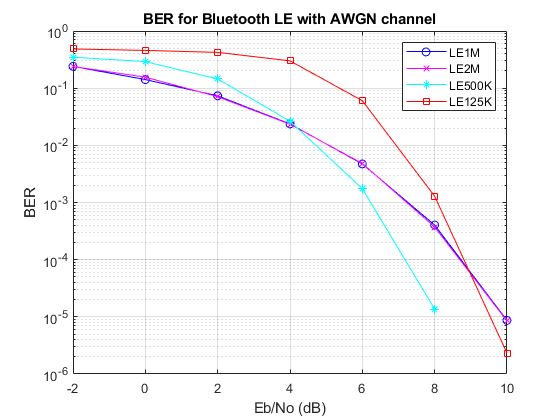# Bluetooth LE Bit Error Rate Simulation with AWGN

This example shows how to measure the bit error rate (BER) for different modes of Bluetooth® low energy (LE) using an end-to-end physical layer simulation by using the Bluetooth® Toolbox.

### Introduction

In this example, an end-to-end simulation is used to determine the BER performance of Bluetooth LE under an additive white Gaussian noise (AWGN) channel for a range of bit energy to noise density ratio (Eb/No) values. At each Eb/No point, multiple Bluetooth LE packets are transmitted through a noisy channel with no other radio front-end (RF) impairments. Assuming perfect synchronization, an ideal receiver is used to recover the data bits. These recovered data bits are compared with the transmitted data bits to determine the BER. BER curves are generated for the four PHY transmission throughput modes supported in Bluetooth LE specification [ 2 ] as follows:

• Uncoded PHY with data rate of 1 Mbps (LE1M)

• Uncoded PHY with data rate of 2 Mbps (LE2M)

• Coded PHY with data rate of 500 Kbps (LE500K)

• Coded PHY with data rate of 125 Kbps (LE125K)

The following diagram summarizes the simulation for each packet.### Initialize the Simulation Parameters

```EbNo = -2:2:8; % Eb/No range in dB sps = 4; % Samples per symbol dataLen = 2080; % Data length in bits simMode = {'LE1M','LE2M','LE500K','LE125K'}; ```

The number of packets tested at each Eb/No point is controlled by two parameters:

1. `maxNumErrors` is the maximum number of bit errors simulated at each Eb/No point. When the number of bit errors reaches this limit, the simulation at this Eb/No point is complete.

2. `maxNumPackets` is the maximum number of packets simulated at each Eb/No point and limits the length of the simulation if the bit error limit is not reached.

The numbers chosen for `maxNumErrors` and `maxNumPackets` in this example will lead to a very short simulation. For statistically meaningful results we recommend increasing these numbers.

```maxNumErrors = 100; % Maximum number of bit errors at an Eb/No point maxNumPackets = 10; % Maximum number of packets at an Eb/No point ```

### Simulating for Each Eb/No Point

This example also demonstrates how a `parfor` loop can be used instead of the `for` loop when simulating each Eb/No point to speed up a simulation. `parfor`, as part of the Parallel Computing Toolbox, executes processing for each Eb/No point in parallel to reduce the total simulation time. To enable the use of parallel computing for increased speed, comment out the 'for' statement and uncomment the 'parfor' statement below. If Parallel Computing Toolbox™ is not installed, 'parfor' will default to the normal 'for' statement.

```numMode = numel(simMode); % Number of modes ber = zeros(numMode,length(EbNo)); % Pre-allocate to store BER results for iMode = 1:numMode phyMode = simMode{iMode}; % Set signal to noise ratio (SNR) points based on mode % For Coded PHY's (LE500K and LE125K), the code rate factor is included % in SNR calculation as 1/2 rate FEC encoder is used. if any(strcmp(phyMode,{'LE1M','LE2M'})) snrVec = EbNo - 10*log10(sps); else codeRate = 1/2; snrVec = EbNo + 10*log10(codeRate) - 10*log10(sps); end % parfor iSnr = 1:length(snrVec) % Use 'parfor' to speed up the simulation for iSnr = 1:length(snrVec) % Use 'for' to debug the simulation % Set random substream index per iteration to ensure that each % iteration uses a repeatable set of random numbers stream = RandStream('combRecursive','Seed',0); stream.Substream = iSnr; RandStream.setGlobalStream(stream); % Create an instance of error rate errorRate = comm.ErrorRate('Samples','Custom','CustomSamples',1:(dataLen-1)); % Loop to simulate multiple packets numErrs = 0; numPkt = 1; % Index of packet transmitted while numErrs < maxNumErrors && numPkt < maxNumPackets % Generate Bluetooth LE waveform txBits = randi([0 1],dataLen,1,'int8'); % Data bits generation channelIndex = randi([0 39],1,1); % Random channel index value for each packet if channelIndex <=36 % Random access address for data channels % Ideally, this access address value should meet the requirements specified in % Section 2.1.2, Part-B, Vol-6 of Bluetooth specification. accessAddress = [1 0 0 0 1 1 1 0 1 1 0 0 1 ... 0 0 1 1 0 1 1 1 1 1 0 1 1 0 1 0 1 1 0]'; else % Default access address for periodic advertising channels accessAddress = [0 1 1 0 1 0 1 1 0 1 1 1 1 1 0 1 1 0 0 ... 1 0 0 0 1 0 1 1 1 0 0 0 1]'; end txWaveform = bleWaveformGenerator(txBits,'Mode',phyMode,... 'SamplesPerSymbol',sps,... 'ChannelIndex',channelIndex,... 'AccessAddress',accessAddress); % Pass the transmitted waveform through AWGN channel rxWaveform = awgn(txWaveform,snrVec(iSnr)); % Recover data bits using ideal receiver rxBits = bleIdealReceiver(rxWaveform,'Mode',phyMode,... 'SamplesPerSymbol',sps,... 'ChannelIndex',channelIndex); % Determine the BER errors = errorRate(txBits,rxBits); ber(iMode,iSnr) = errors(1); numErrs = errors(2); numPkt = numPkt + 1; end disp(['Mode ' phyMode ', '... 'Simulating for Eb/No = ', num2str(EbNo(iSnr)), ' dB' ', '... 'BER:',num2str(ber(iMode,iSnr))]) end end ```
```Mode LE1M, Simulating for Eb/No = -2 dB, BER:0.23617 Mode LE1M, Simulating for Eb/No = 0 dB, BER:0.16162 Mode LE1M, Simulating for Eb/No = 2 dB, BER:0.07696 Mode LE1M, Simulating for Eb/No = 4 dB, BER:0.023088 Mode LE1M, Simulating for Eb/No = 6 dB, BER:0.0050238 Mode LE1M, Simulating for Eb/No = 8 dB, BER:0.00042756 Mode LE2M, Simulating for Eb/No = -2 dB, BER:0.24627 Mode LE2M, Simulating for Eb/No = 0 dB, BER:0.17268 Mode LE2M, Simulating for Eb/No = 2 dB, BER:0.071188 Mode LE2M, Simulating for Eb/No = 4 dB, BER:0.025493 Mode LE2M, Simulating for Eb/No = 6 dB, BER:0.0044893 Mode LE2M, Simulating for Eb/No = 8 dB, BER:0.00074822 Mode LE500K, Simulating for Eb/No = -2 dB, BER:0.35546 Mode LE500K, Simulating for Eb/No = 0 dB, BER:0.29197 Mode LE500K, Simulating for Eb/No = 2 dB, BER:0.1443 Mode LE500K, Simulating for Eb/No = 4 dB, BER:0.026615 Mode LE500K, Simulating for Eb/No = 6 dB, BER:0.00080167 Mode LE500K, Simulating for Eb/No = 8 dB, BER:0 Mode LE125K, Simulating for Eb/No = -2 dB, BER:0.35161 Mode LE125K, Simulating for Eb/No = 0 dB, BER:0.066859 Mode LE125K, Simulating for Eb/No = 2 dB, BER:0.0023516 Mode LE125K, Simulating for Eb/No = 4 dB, BER:0 Mode LE125K, Simulating for Eb/No = 6 dB, BER:0 Mode LE125K, Simulating for Eb/No = 8 dB, BER:0 ```

```markers = 'ox*s'; color = 'bmcr'; dataStr = {zeros(numMode,1)}; figure; for iMode = 1:numMode semilogy(EbNo,ber(iMode,:).',['-' markers(iMode) color(iMode)]); hold on; dataStr(iMode) = simMode(iMode); end grid on; xlabel('Eb/No (dB)'); ylabel('BER'); legend(dataStr); title('BER for Bluetooth LE with AWGN channel'); ```### Further Exploration

The number of packets tested at each Eb/No point is controlled by `maxNumErrors` and `maxNumPackets` parameters. For statistically meaningful results these values should be larger than those presented in this example. The figure below was created by running the example for longer with `maxNumErrors = 1e3`, `maxNumPackets = 1e4`, for all the four modes.### Summary

This example simulates a Bluetooth LE physical layer link over an AWGN channel. It shows how to generate Bluetooth LE waveforms, demodulate and decode bits using an ideal receiver and compute the BER.

### Selected Bibliography

1. Bluetooth Technology Website | The Official Website of Bluetooth Technology, Accessed November 22, 2021. https://www.bluetooth.com.

2. Volume 6 of the Bluetooth Core Specification, Version 5.3 Core System Package [Low Energy Controller Volume].